## Roulettes, non-Russian

2017 July 3

As stated in the title, I'm not talking about a game of chance that you play with old-fashioned pistols. Nor am I talking about the casino game. In the mathematics of curves, a roulette is what you get when you roll one curve along another, and trace a point on or inside of the curve as it is rolled. One example of a roulette is a cycloid, which is obtained by rolling a circle along a straight line and tracing a point on its circumference. This is what a cycloid looks like: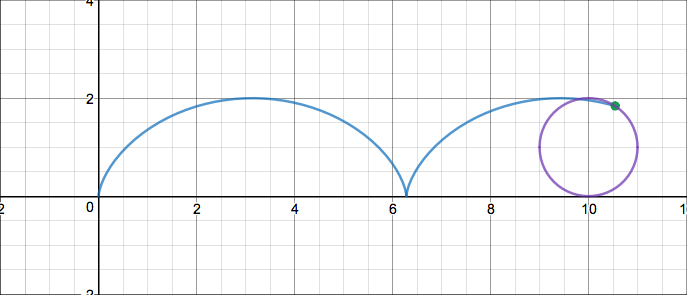We will be deriving the parametric equation for the cycloid, as well as the parametric equations for many other interesting roulettes. But first, something a little more basic. We will start with the roulettes formed by rolling a regular polygon along a line. These are a good place to start, as they are much easier to work with than some other roulettes. The problem to which we will be turning is the length of the path traced by a vertex of a polygon in one "cycle" of its roulette.

The way that we would generate such a roulette is by placing a regular polygon of side length $l$ into the coordinate plane so that the point which we are tracing is on top of the origin and one side of the polygon containing the tracing point is laid over the $x$-axis, covering the length from $x=0$ to $x=l$. Then, to roll the polygon, we would "pivot" it about its rightmost point resting on the $x$-axis until another point touches the $x$-axis, and then we would pivot about that one, and so on, until all vertices of the polygon have been "pivoted about". Here are the pictures of the cycloids formed by an equilateral triangle, a square, and a regular pentagon: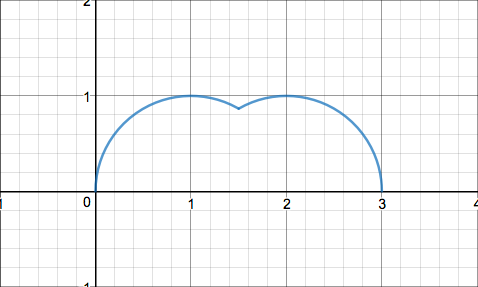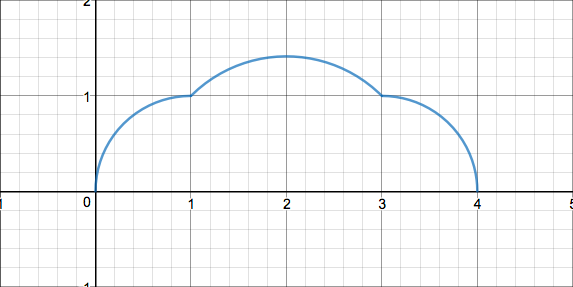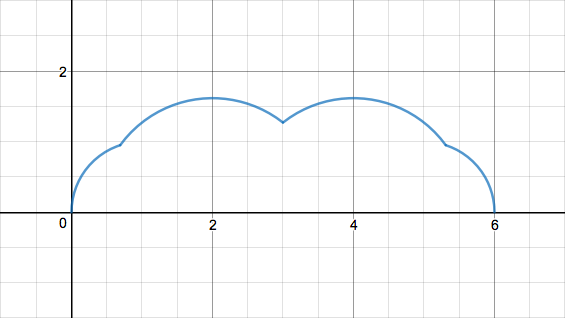This is more of a geometry problem than anything else, other than a partial sum involving trigonometric functions that we will have to evaluate. As you can see from the pictures, each $n$-gonal cycloid is basically composed of $n-1$ circle sectors attached to each other. A bit of further observation reveals that each circle sector measures $\frac{2\pi}{n}$ radians.

First let's just calculate the length for the triangular cycloid as an easy example. We can see that the angle of each sector is $\frac{2\pi}{3}$ radians, and the radius of each one is equal to the side length of the triangle, the length of the total path is

Now for the square. This time we have three sectors. The radii of the two smaller ones are equal to the side length $l$ of the square, and the radius of the larger one is equal to the diagonal length of the square, or $l\sqrt 2$. The angle of each sector is $\frac{\pi}{2}$, and so the total length is

Now we are ready to generalize. Since each circle is formed by rotating one vertex of the polygon (namely, the tracing point) about another, then the radii of the circles are equal to the lengths of the diagonals of the polygon. Thus the total length is equal to We can extract the constant factor from the summation and simplify it to Where $d_x$ is the length of the xth diagonal counterclockwise from the tracing point. If we can find $d_x$ as a function of $x$ and $l$, then we may be able to simplify our sum even further.

Using a bit of geometry, we can find that the angle formed by joining the tracing point to the center of the polygon and the xth vertex counterclockwise measures $\frac{2\pi x}{n}$ radians. Furthermore, if we join the tracing point to that vertex to form the xth diagonal, we then have a isocscles triangle with angle $\frac{2\pi x}{n}$ opposite its base and two congruent sides that join the center of the polygon to two different vertices of the polygon. Again, using a bit of geometry, we can find that the length of the line segment joining the center of a regular $n$-gon with side length $l$ is given by and so now we have an isoscles triangle measuring as shown, and we need to find the base length: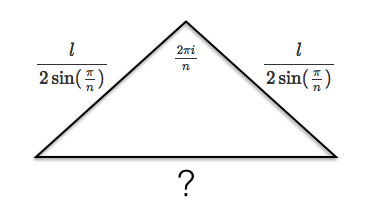I'll spare you the details of the rest of the geometry. It turns out that the diagonal length turns out the be

and that means that we can now transform our sum into

Luckily, we learned how to evaluate this type of sum in my post regarding non-telescoping sums. The value of the summation is equal to the imaginary part of And now we can readily sum these terms using the formula used for the sum of the terms of a geometric sequence: now we just need to find the imaginary part. and so the imaginary part is We can now simplify this down a little bit:

And so the length of the path traced is

Now onto something a bit more interesting. We will now derive the parametric equation of a cycloid.

A cycloid is typically formed by a circle with radius $r$ starting with its center at $(0,r)$ and the tracing point at the origin. There is one further given that we must accept - the idea that the length of the segment of the $x$-axis rolled over by the circle at some time $t$ is always equal to the arclength of the circle that has been rolled on. This is fundamental to the concept of roulettes in general, and this law can be generalized by replacing the statement "segment of the $x$-axis" with "segment of the curve rolled on" and "circle" with "rolling curve".

Okay, time to derive the parametric equation! Let $r$ be radius of the circle and let the circle turn $1$ radian per unit of time, so that the total angle that it has turned is $t$. Thus we can construct the following diagram for any time $t$: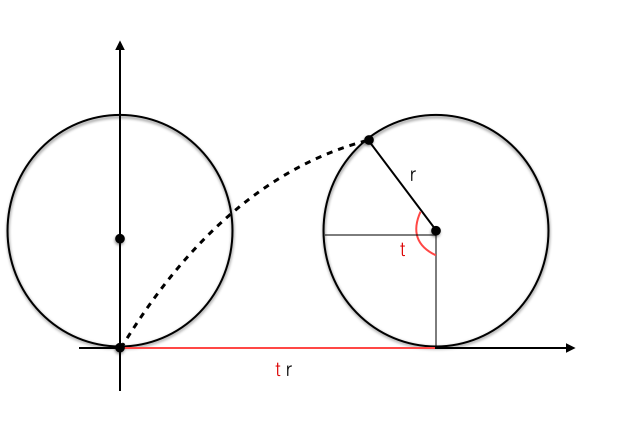Before we derive the parametric equation for the cycloid using this diagram, I would like to say a word about a method that I call "relative coordinates". Often, when dealing with tranformations and parametric equations, this strategy can be very useful. Here is an example of how it is used.

Suppose we want to find the image of the point $(3,3)$ dilated about the point $(2,5)$ by a factor of $2$. It is very tiresome to dilate points about points, but if we were dilating about the origin, it would be easy - we would just have to multiply the coordinates by two! So that is what we will do. We will translate both points in such a way that $(2,5)$ maps onto the origin, and then we will proceed with the dilation, and then we will move $(2,5)$ back to its original position. Thus we will translate both points $2$ units left and $5$ units down, moving $(2,5)$ to $(0,0)$ and $(3,3)$ to $(1,-2)$. Now we can dilate, and now we need only double the abscissa and ordinate of $(1,-2)$ to get $(2,-4)$. Now we translate everything back to where it was before, by shifting both points right $2$ units and back up $5$ units. $(0,0)$ goes back to $(2,5)$ and our dilated point $(2,-4)$ becomes $(4,1)$. That wasn't so bad!

We can use the same process for parametric equations. It is often overcomplicated to go directly to finding the coordinates of the point that we wish to trace. We must often first choose some "reference point" that has coordinates that are simpler to find. Then if we find the coordinates of the reference point as a function of $t$ and then find the relationship between the reference point and the tracing point, we can "combine" these to get our final parametric equation.

Time to derive the parametric equation of the cycloid! Here's our beautiful diagram again:From the diagram, we can see that an obvious reference point would be the center of the rolling circle. The center of the circle obviously has the coordinates Now we need to find the coordinates of the tracing point relative to the center of the circle - that is, what the coordinates of the tracing point would be if the center of the circle was always at the origin. If we suppose that the center of our circle is at the origin, then we get something like this: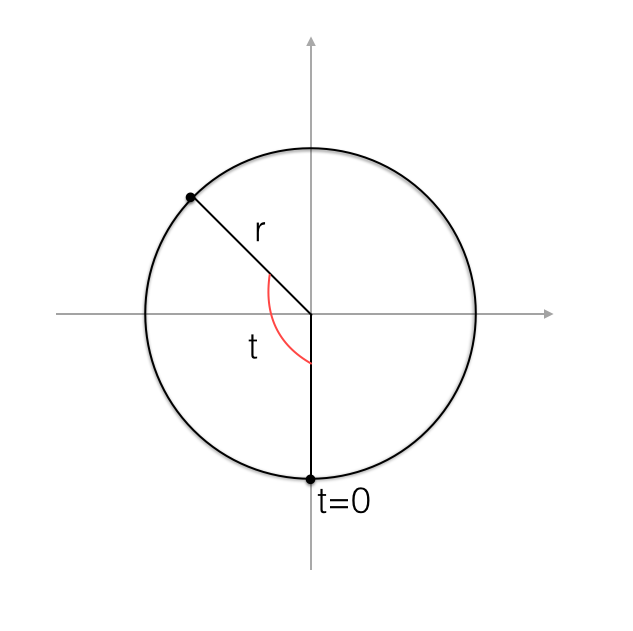This should be familiar to you - it's basically the unit circle dilated by a factor of $r$!

As you should know, the parametric equation for the normal circle (starting with a point at $(r,0)$ and then rotating counterclockwise) is but our circle is a little different. It starts at $(0,-r)$ and travels clockwise rather than counterclockwise. Thus the angle at which it starts is $\frac{\pi}{2}$ less than the starting angle of the normal circle. Furthermore, since it is rotating clockwise rather than counterclockwise, the $x$ coordinate of the point is reflected. Thus the parametric equation of our special circle is or When we came up with this, we were treating the center of our circle as if it was the origin. Now we must translate it back to $(rt,r)$, and, in the process, translate our tracing point to And this is it - this is the parametric equation for a cycloid! We did it!

Now we shall find the length of one revolution of the cycloid, from $t=0$ to $t=2\pi$.

But wait... that would require us to evaluate a messy integral of a parametric equation. There's a much easier way to do this using a formula that we have already derived: the formula for the length of the path traced by a polygon rather than a circle. We can also use the fact that as a polygon with apothem $r$ gains more and more sides, it approaches a circle with radius $r$. So suppose a polygon has apothem $r$ and $n$ sides. Then (using a little geometry) we can say that the side length of the polygon is and so we can use our previous formula to say that the length of a cycloid is If we make the substitution $n \to \frac{1}{n}$, we get And since $\lim_{n \to 0} \sec(n \pi)=1$, we have And with the substitution $n \to \frac{2}{\pi}n$, we have

And so the length of one revolution of a cycloid is $8$ times the radius of the circle that generates it. What a beautiful property!

Now it is time for something trickier - the hypocycloid. This is slightly different from the regular cycloid. It still features a rolling circle... but the circle now rolls along another larger circle, rather than along a line. In a hypocycloid, a smaller circle rolls along the inside of a larger one: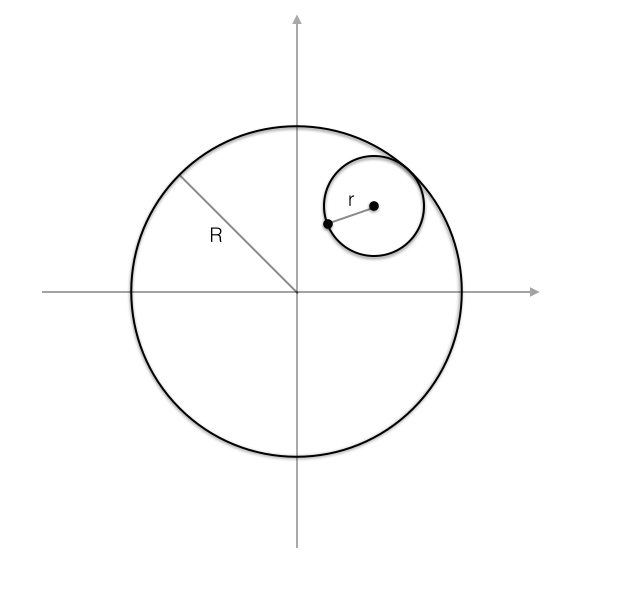Let $t$ be the amount of arclength rolled on by the smaller circle, and let $\alpha$ be the angle formed by joining the origin to both the center of the rolling circle and the point at which its center started. Then the amount of arclength rolled over on the large one must be equal to the arclength rolled on by the circle, which means that and Now, as a reference point to the tracing point, we can use the center of the small circle. When traced, it forms a circle of radius $R-r$, which is easy to see, and since the angle that it makes with the origin and the starting position is $\alpha$, we have that its coordinates are or and, relative to the center of the small circle (if it was the origin), the relative coordinates of the tracing point are or and so, when we translate the origin back to the center of the small circle where it should be, the coordinates of the tracing point become

And this is it - the general parametric equation for a hypocycloid. Here are some pictures of hypocycloids: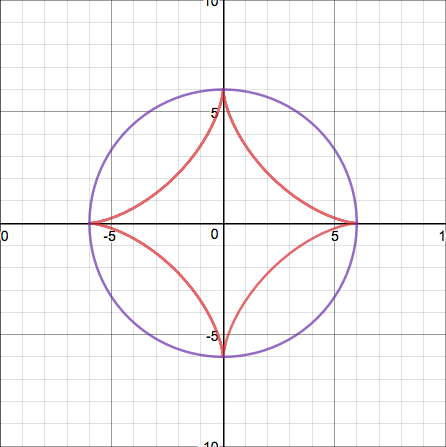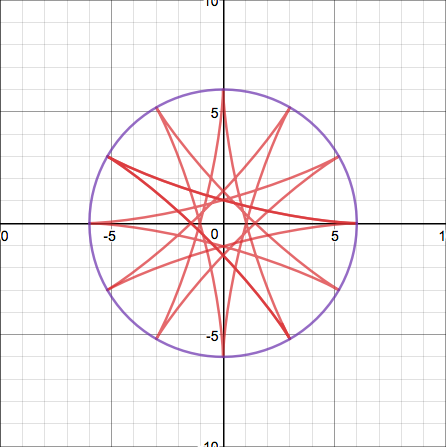The shape made by the first is called a deltoid, the shape made by the second is called an astroid, and the third is just a random cool-looking hypocycloid.

Just one more thing before I end this blog post.

We've been working with rolling circles so far, but now I'd like to show you what happens when we try to roll a line along another curve, and trace a point on it. The curve generated by this process is called the involute of the curve along which the line is rolled.

How would this work? Basically, if we were rolling a line along the curve $y=f(x)$, we would let $t$ be the $x$ coordinate at which the line touches the curve. Then the equation of the line would basically just be the tangent to the curve at that point, and the tracing point would appear some length $L$ away from the point of tangency along the line, where $L$ is the length of the curve from $x=0$ to $x=t$. Recall the formula for the arclength of a curve from $x=0$ to $x=t$:

and so we can construct the following diagram: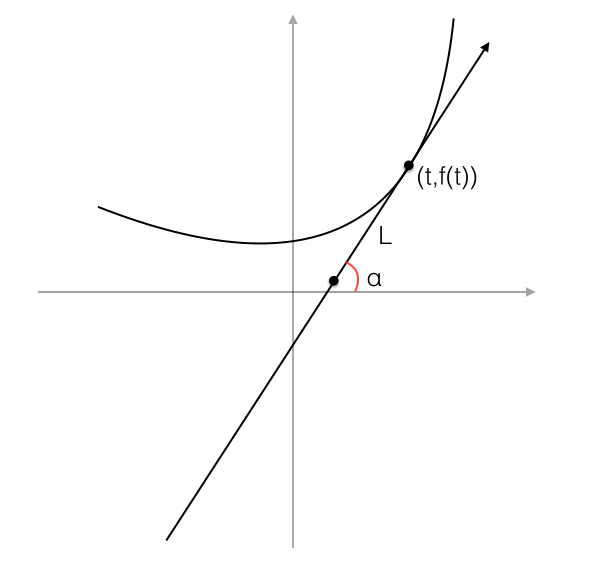We can see that the slope of the tangent line is $f'(t)$ and so its angle of elevation is $\arctan f'(t)$. This means that, if we let the tracing point be $(x,y)$, we can construct a right triangle and say that and

And that's it! Our parametric equation is

Here are a few interesting curve involutes. The original curves are in red and their involutes are in blue. They are, respectively, a parabola, an exponential function, and a sine wave: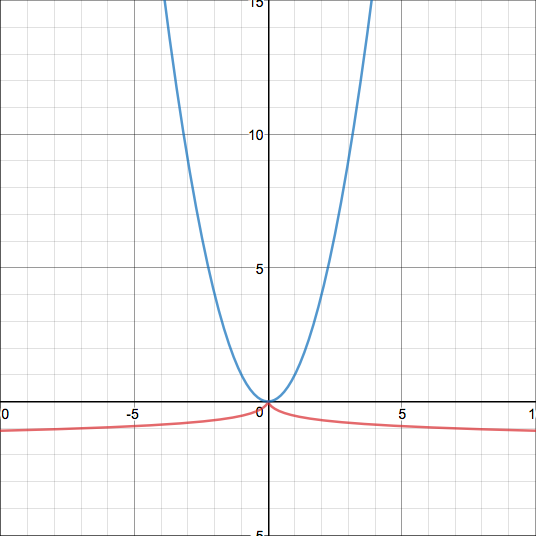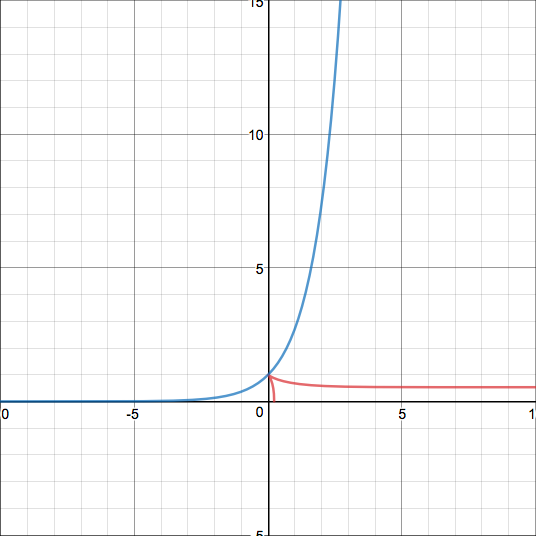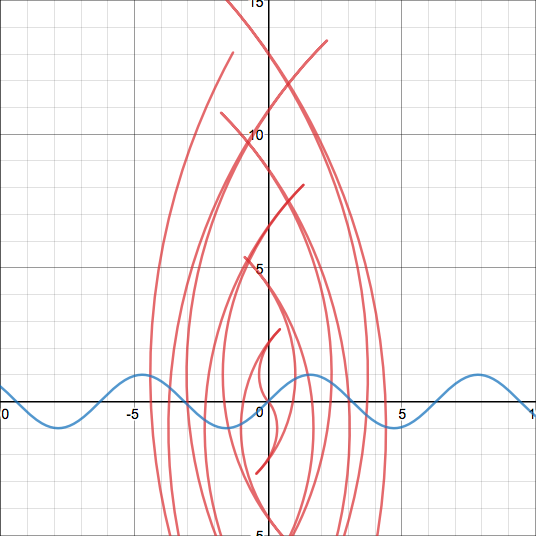If you want to play around with this a little more, I have created, at desmos.com, a graph in which you can input any function whose involute you wish to see, and there you can watch the line roll along it, which should help with any trouble understanding what it means for a line to "roll" along a curve. You can find it at this link. Have fun!

Well, that concludes this post. I will definitely return to this topic later, though - there is much more to learn about the involutes of curves. For examples, our formula does not apply to parametric equations, who also have involutes. Furthermore, you don't just have to roll lines along curves - you can roll curves along other curves. But I guess I'll just have to put that off until later and end this post with a cliff hanger...